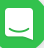Only Highly Intelligent People Can Pass This IQ TEST - Quiz - Emmanuel's Blog.....Expect Everything New, Inspiring, DIY, Fab & Projecting Vibrant posibility of Africa

## 2/15/2020

#### HOW SMART ARE YOU?

The word I. Q is not new to anyone, but still, some people do not know its meaning. The word I. Q stands for Intelligent Quotient.

I. Q simply refers to your level of intelligence and reasoning. Everyone has different levels of I. Q. But the main thing however, is it's level. A person with a high level of I. Q is proven to be very smart intellectually, with a high level of reasoning.

How does one test their I. Q? You do so by taking various tests to determine whether or not you're I. Q level is high. One of such test is shown in the image below.

Take a look at the image above. It shows a series of images and numbers. Tricky as this may be, it is meant to awaken your interest and make you think.
In the first row of the image, 3 pairs of earbuds are said to be equal to 30. This means each pair is equal to 10.

In the second row, we see the same pair of earbuds that we have already established as 10, together with two icons of a person, and the sum of the whole thing is 20. To find the numeral representation of the person, we subtract that of the earbuds from the final score, and that gives us a value of 10. This means each icon of the person represents the number 5.

The third row, shows a person, and two pairs of watches. We already know that the person equals 5, and to find the value of the pairs, we subtract 5 from 13. The value is 8,and this means each icon of a pair of watch represents 4.

Finally, the final row, is where the test really lies. We have been able to determine the value of each icon.
Each pair of earbuds =10. (Each earbud in a pair is 5)
Each person icon =5
Each pair of watch =4 ( each watch in a pair is 2)

To find the missing value of the last row, we take all these things to note.
One half of a pair of earbud is 5

The person icon is 5, but when you look closely, you will discover that the person icon wears a watch and a pair of earbud. This means the person icon(5) + a pair of earbuds(10) + one watch (2). This totals 17.

Then one half of a pair of watch is 2.
The final value can be gotten by
5 + ( 5+ 10+ 2) × 2 =?
5 + 17 × 2 =?
5+ 34 = 39
The final value is 39
##### Do You like our post? Make sure you never miss any of it! Follow us on Twitter it will encourage us alot Follow @EmmanuelsblogTV on Twitter
11.